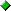WEB SITE NEWS
Formula List of Algebra, Geometry, Trigonometry, and Calculus

 AlgebraAddition TableMultiplication TableRules for AdditionMath SymbolsUnit Conversion (length)Unit Conversion (mass)Unit Conversion (area)Units Conversion (volume)Negative Numbers AdditionRules for FractionTypes of NumbersNames of Big NumbersRoman NumeralsPrime NumberArithmetic Operations on FunctionsSquares and Square Roots of IntegersDecimal, Binary and Hexadecimal Number ConversionAbsolute ValueBasic IdentitiesExponentsFactorialsConsecutive IntegersPolynomial Vocabulary

 GeometryPolygon NameAreaVolumePerimeter

 TrigonometryTrigonometric FormulaTrigonometric FunctionTrigonometric GraphsTrigonometric Properties (I)Trigonometric Properties (II)Trigonometric Properties (III)

 CalculusDerivativesIntegrals